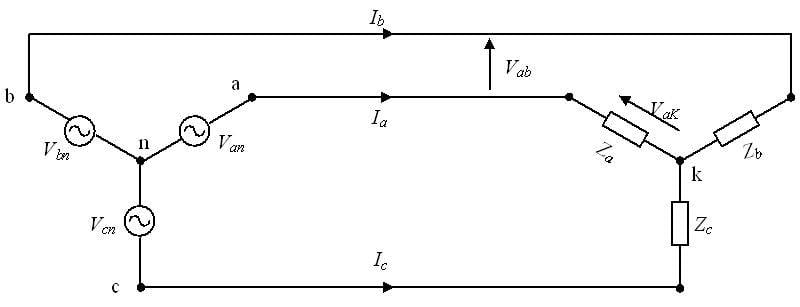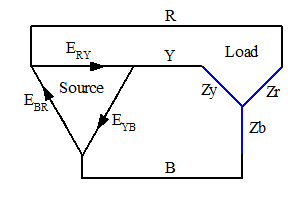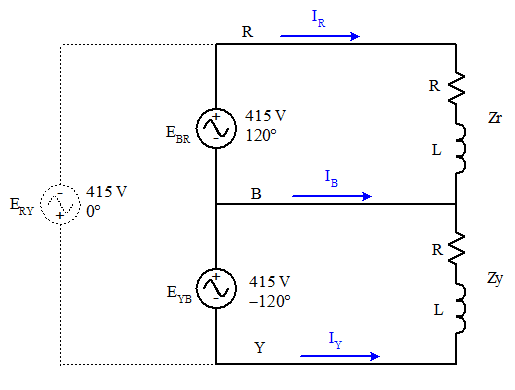# Short Circuiting 1 of 3 coils that are Wye connected

• Engineering
For this question i'm focusing on part B

1. Homework Statement

Three similar coils, connected in star, take a total power of 1.5 kW, at a power factor of 0.2, from a three-phase, 415 V, 60 Hz supply. Calculate:

a) the resistance and inductance of each coil;

b) the line currents if one of the coils is short circuited.

## The Attempt at a Solution

If I have say three coils that are in a star arrangement and are supplied to by a 3 phase power supply like below (the power supply could be star/delta arranged but i'm just using this pic for illustration to aid the question)If I were to short circuit one of the coils say...Zc (which is equivalent to R+jXl for this example...as would Za and Zb as they're all similar coils)...what would happen to the system???

I'm assuming that the line currents remain unchanged as they're supplied by the source. But what about the line voltages?
Would the line voltage Vca be equal to Vak in this case...and Vab remain unchanged?

gneill
Mentor
I'm assuming that the line currents remain unchanged as they're supplied by the source. But what about the line voltages?
If you take the power supply to be comprised of ideal voltage sources then the line-to-line voltages must remain the same. It's the currents that can change.
Would the line voltage Vca be equal to Vak in this case...and Vab remain unchanged?
Yes.

Does that then mean that Vak would then be 415V and Line current = 1.73*phase current where the phase current is 415/|Z|?

gneill
Mentor
Does that then mean that Vak would then be 415V and Line current = 1.73*phase current where the phase current is 415/|Z|?

Please show your analysis of the circuit. Keep in mind that when you break the symmetry of a Δ or Y load configuration (such as by shorting out one of its components so that it is no longer balanced), the standard equations that assume symmetry may no longer hold. That would mean going back to basics to analyze the circuit.

This was my attemp at it using circuit analysis

#### Attachments

gneill
Mentor
It looks like your phasor algebra is on track. Not being three-phase power systems guru I must admit though that going right to phasor diagrams is not my favorite approach. Let's see if it can be confirmed by a basic circuit analysis approach.

Okay, so with one of the loads shorted you're left with two loads with 415 V across each. They are effectively independent with separate voltage sources driving them, but share one of the phase wires. Let's see how we can draw the resulting circuit in a way that's convenient for analysis.

Consider a general setup where a Y load is being driven by a Δ source:The Δ source voltages ##E_{RY}##, ##E_{YB}##, and ##E_{BR}## supply the line-to-line 415 V potential differences. Zr, Zy, and Zb are the individual load impedances. You should be able to assign the phase angles to each of the source supplies; just start at 0° and subtract 120° for each as you go around the Δ of sources (to preserve the sequence R-Y-B for the phase voltages). What's important is that the appropriate relative phases are preserved.

If load Zb becomes shorted then that will put source ##E_{YB}## directly across load Zy, and source ##E_{BR}## directly across Zr. Assuming that the sources are ideal, nothing is going to influence their voltages and phase angles. So those loads are going to be driven by those supplies and we can ignore the ##E_{RY}## source. We're looking for the line currents for the lines R, Y, and B.

Rearrange the diagram to place the B line in the middle (since it's become a convenient "common" node for the two remaining loads thanks to Zb being shorted). Keep track of the source phases and directions! Note also that -240° is the same as +120°. We have:That should be easy enough to solve for the currents.

•cnh1995
Woa thanks man.
Our lecturer stresses so much on phasors all the time that it was the first thing that came to me.

Ill work it using both methods and post my soln

Okay so I worked them both ways and i'm encountering a problem.

Using the formula I derived for Ib from the phasor diagram I substituted Iy & Ir = 1.8346A which I obtained from Ir(or Iy) = 415/|Z|
where Z = R+jXl i.e Z = sqrt(R^2+(omega*f)^2)

I previously calculated R as 47.17ohms and L as 0.59H

This resulted in me getting Ib as 1.8346A as well.

However using your method I attempted it using Mesh analysis

For Mesh 1:
415<120= -I1*Zr
I1 = 1.8346<-60 where I1 = Ir

For Mesh 2
415<-120 =-I2*Zy
I2 = 1.8346<60 where Iy = -I2 i.e Iy = 1.8346<-120

Ib = I2-I1
= 1.8346<60-1.8346<-60 = 3.177<90

Could this be because I worked the question considering it was a Y connected source?

#### Attachments

gneill
Mentor
Y or Δ source shouldn't matter if you translated the source voltages accordingly.

You didn't present how you arrived at the resistor and inductor values. Did you check your results by confirming the real power that they consume in the original circuit?

gneill
Mentor
If I calculate the power consumed in the original circuit using your component values I'm only seeing a total of about 160 W, not the expected 1.5 kW.

A Y-configured source would have source voltages of about 240 V. (That's 415 V / √3). For a balanced network each of the Y sources drives one leg of the Y load (the center node is a virtual ground). So for a total dissipation of 1500 W each leg should dissipate 500 W.

With your component values, Z = (47.17 + j222.4) Ω. Then for one leg:

##I = \frac{240~ V}{Z} = (0.219 - j1.033)~ A##

##P = (240~ V)(I^*) = (240~ V)(0.219 + j1.033)~ A = (52.4 + j247)~VA##

So only about 52 watts per leg.